# Stein Unbiased Risk Estimator¶

Important: Please read the installation page for details about how to install the toolboxes. $\newcommand{\dotp}{\langle #1, #2 \rangle}$ $\newcommand{\enscond}{\lbrace #1, #2 \rbrace}$ $\newcommand{\pd}{ \frac{ \partial #1}{\partial #2} }$ $\newcommand{\umin}{\underset{#1}{\min}\;}$ $\newcommand{\umax}{\underset{#1}{\max}\;}$ $\newcommand{\umin}{\underset{#1}{\min}\;}$ $\newcommand{\uargmin}{\underset{#1}{argmin}\;}$ $\newcommand{\norm}{\|#1\|}$ $\newcommand{\abs}{\left|#1\right|}$ $\newcommand{\choice}{ \left\{ \begin{array}{l} #1 \end{array} \right. }$ $\newcommand{\pa}{\left(#1\right)}$ $\newcommand{\diag}{{diag}\left( #1 \right)}$ $\newcommand{\qandq}{\quad\text{and}\quad}$ $\newcommand{\qwhereq}{\quad\text{where}\quad}$ $\newcommand{\qifq}{ \quad \text{if} \quad }$ $\newcommand{\qarrq}{ \quad \Longrightarrow \quad }$ $\newcommand{\ZZ}{\mathbb{Z}}$ $\newcommand{\CC}{\mathbb{C}}$ $\newcommand{\RR}{\mathbb{R}}$ $\newcommand{\EE}{\mathbb{E}}$ $\newcommand{\Zz}{\mathcal{Z}}$ $\newcommand{\Ww}{\mathcal{W}}$ $\newcommand{\Vv}{\mathcal{V}}$ $\newcommand{\Nn}{\mathcal{N}}$ $\newcommand{\NN}{\mathcal{N}}$ $\newcommand{\Hh}{\mathcal{H}}$ $\newcommand{\Bb}{\mathcal{B}}$ $\newcommand{\Ee}{\mathcal{E}}$ $\newcommand{\Cc}{\mathcal{C}}$ $\newcommand{\Gg}{\mathcal{G}}$ $\newcommand{\Ss}{\mathcal{S}}$ $\newcommand{\Pp}{\mathcal{P}}$ $\newcommand{\Ff}{\mathcal{F}}$ $\newcommand{\Xx}{\mathcal{X}}$ $\newcommand{\Mm}{\mathcal{M}}$ $\newcommand{\Ii}{\mathcal{I}}$ $\newcommand{\Dd}{\mathcal{D}}$ $\newcommand{\Ll}{\mathcal{L}}$ $\newcommand{\Tt}{\mathcal{T}}$ $\newcommand{\si}{\sigma}$ $\newcommand{\al}{\alpha}$ $\newcommand{\la}{\lambda}$ $\newcommand{\ga}{\gamma}$ $\newcommand{\Ga}{\Gamma}$ $\newcommand{\La}{\Lambda}$ $\newcommand{\si}{\sigma}$ $\newcommand{\Si}{\Sigma}$ $\newcommand{\be}{\beta}$ $\newcommand{\de}{\delta}$ $\newcommand{\De}{\Delta}$ $\newcommand{\phi}{\varphi}$ $\newcommand{\th}{\theta}$ $\newcommand{\om}{\omega}$ $\newcommand{\Om}{\Omega}$

This tour uses the Stein Unbiased Risk Estimator (SURE) to optimize the value of parameters in denoising algorithms.

In :
from __future__ import division

import numpy as np
import scipy as scp
import pylab as pyl
import matplotlib.pyplot as plt

from nt_toolbox.general import *
from nt_toolbox.signal import *

import warnings
warnings.filterwarnings('ignore')

%matplotlib inline


## Denoising and SURE¶

We consider a simple generative model of noisy images $F = f_0+W$ where $f_0 \in \RR^N$ is a deterministic image of $N$ pixels, and $W$ is a Gaussian white noise distributed according to $\Nn(0,\si^2 \text{Id}_N)$, where $\si^2$ is the variance of noise.

The goal of denoising is to define an estimator $h(F)$ of $f_0$ that depends only on $F$, where $h : \RR^N \rightarrow \RR^N$ is a potentially non-linear mapping.

Note that while $f_0$ is a deterministic image, both $F$ and $h(F)$ are random variables (hence the capital letters).

The goal of denoising is to reduce as much as possible the denoising error given some prior knowledge on the (unknown) image $f_0$. A mathematical way to measure this error is to bound the quadratic risk $\EE_W(\norm{h(F) - f_0}^2)$, where the expectation is computed with respect to the distribution of the noise $W$

For real life applications, one does not have access to the underlying image $f_0$. In this tour, we however assume that $f_0$ is known, and $f = f_0 + w\in \RR^N$ is generated using a single realization of the noise $w$ that is drawn from $W$. We define the estimated deterministic image as $h(f)$ which is a realization of the random vector $h(F)$.

Number $N = n \times n$ of pixels.

In :
n = 128*2
N = n**2


First we load an image $f \in \RR^N$ where $N=n \times n$ is the number of pixels.

In :
f0 = load_image("nt_toolbox/data/hibiscus.bmp",n)


Display it.

In :
plt.figure(figsize = (5,5))
imageplot(f0)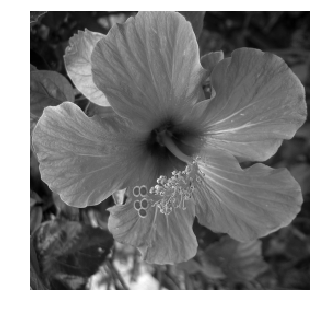Standard deviation $\si$ of the noise.

In :
sigma = .08


Then we add Gaussian noise $w$ to obtain $f=f_0+w$.

In :
from numpy import random
f = f0 + sigma*random.standard_normal((n,n))


Display the noisy image. Note the use of the clamp function to force the result to be in $[0,1]$ to avoid a loss of contrast of the display.

In :
plt.figure(figsize = (5,5))
imageplot(clamp(f), "Noisy, SNR = %.1f dB" %snr(f0, f))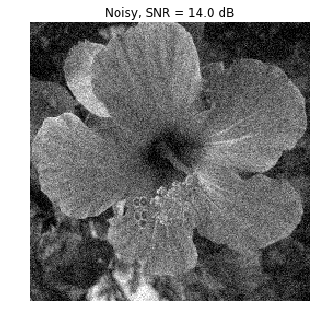The Stein Unbiased Risk Estimator (SURE) associated to the mapping $h$ is defined as

$$\text{SURE}(f) = -N\si^2 + \norm{h(f)-f}^2 + 2\si^2 \text{df}(f)$$

where df stands for degree of freedom, and is defined as

$$\text{df}(f) = \text{div} h(f) = \sum_i \pd{h}{f_i}(f).$$

It has been introduced in:

Stein, Charles M. (November 1981). "Estimation of the Mean of a Multivariate Normal Distribution". The Annals of Statistics 9 (6): 1135-1151.

And it has been applied to wavelet-based non-linear denoising in:

Donoho, David L.; Iain M. Johnstone (December 1995). "Adapting to Unknown Smoothness via Wavelet Shrinkage". Journal of the American Statistical Association (Journal of the American Statistical Association, Vol. 90, No. 432) 90 (432): 1200-1244.

If the mapping $f \mapsto h(f)$ is differentiable outside a set of zero measure (or more generally weakly differentiable), then SURE defines an unbiased estimate of the quadratic risk :

$$\EE_W(\text{SURE}(F)) = \EE_W( \norm{f_0-h(F)}^2 ).$$

This is especially useful, since the evaluation of SURE does not necessitate the knowledge of the clean signal $f_0$ (but note however that it requires the knowledge of the noise level $\si$).

In practice, one replaces $\text{SURE}(F)$ from its empirical evaluation $\text{SURE}(f)$ on a single realization $f$. One can then minimize $\text{SURE}(f)$ with respect to a parameter $\la$ that parameterizes the denoiser $h=h_\la$.

## Linear Denoising SURE¶

We consider a translation-invariant linear denoising operator, which is thus a convolution

$$h(f) = f \star g$$

where $g \in \RR^N$ is a low pass kernel, and $\star$ denotes the periodic 2-D convolution.

Since we use periodic boundary condition, we compute the convolution as a multiplication over the Fourier domain.

$$\forall \om, \quad \hat h(f)(\om) = \hat f(\om) \hat g(\om)$$

where $\hat g(\om)$ is the frequency $\om$ of the discrete 2-D Fourier transform of $g$ (computed using the pylab function fft2 from the pylab package).

In :
convol = lambda f,g: np.real(pyl.ifft2(pyl.fft2(f)*np.tile(pyl.fft2(g), (1,1))))


We define a parameteric kernel $g_\la$ parameterized by its bandwidth $\la>0$. We use here a Gaussian kernel

$$g_\la(a) = \frac{1}{Z_\la} e^{ -\frac{\norm{a}}{2 \la^2} }$$

where $Z_\la$ ensures that $\sum_a g_\la(a) = 1$.

In :
normalize = lambda f: f/np.sum(f)
x = np.hstack((np.arange(0,n//2+1),np.arange(-n//2 + 1,0)))
[Y, X] = np.meshgrid(x, x)
g = lambda lambd: normalize(np.exp(-(X**2 + Y**2)/(2*lambd**2)))


Define our denoising operator $h=h_\la$ (we make explicit the dependency on $\la$): $$h_\la(f) = g_\la \star f.$$

In :
h = lambda f, lambd: convol(f, g(lambd))


Example of denoising result.

In :
lambd = 1.5

plt.figure(figsize = (5,5))
imageplot(clamp(h(f, lambd)))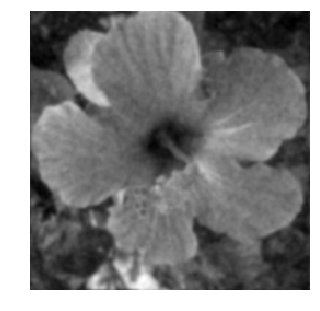For linear operator, the dregree of freedom is equal to the trace of the operator, and thus in our case it is equal to the sum of the Fourier transform $$\text{df}_\la(f) = \text{tr}(h_\la) = \sum_{\om} \hat g_\la(\om)$$ Note that we have made explicit the dependency of df with respect to $\la$. Note also that df$(f)$ actually not actually depend on $f$.

In :
df = lambda lambd: np.real(np.sum(pyl.fft2(g(lambd))))


We can now define the SURE=SURE$_\la$ operator, as a function of $f, h(f), \lambda$.

In :
from numpy import linalg
SURE = lambda f,hf,lambd: -N*sigma**2 + linalg.norm(hf-f,"fro")**2 + 2 * sigma**2 * df(lambd)


Exercise 1

For a given $\lambda$, display the histogram of the repartition of the quadratic error $\norm{y-h(y)}^2$ and of $\text{SURE}(y)$. Compute these repartition using Monte-Carlo simulation (you need to generate lots of different realization of the noise $W$). Display in particular the location of the mean of these quantities.

In :
run -i nt_solutions/denoisingadv_9_sure/exo1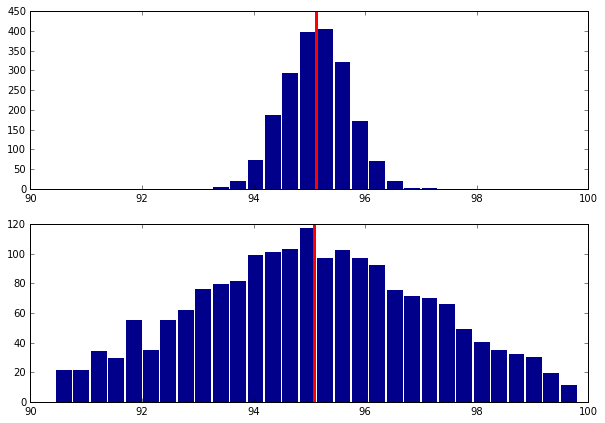In :
## Insert your code here.


In practice, the SURE is used to set up the value of $\la$ from a single realization $f=f_0+w$, by minimizing $\text{SURE}_\la(f)$.

Exercise 2

Compute, for a single realization $f=f_0+w$, the evolution of

$$E(\la) = \text{SURE}_\la(f) \qandq E_0(\lambda) = \norm{f-h_\la(f)}^2$$

as a function of $\lambda$.

In :
run -i nt_solutions/denoisingadv_9_sure/exo2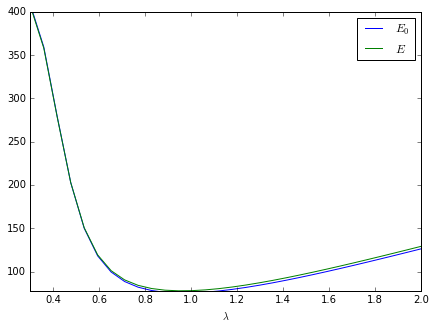In :
## Insert your code here.


Exercise 3

Display the best denoising result $h_{\la^*}(f)$ where $$\la^* = \uargmin{\la} \text{SURE}_\la(f)$$

In :
run -i nt_solutions/denoisingadv_9_sure/exo3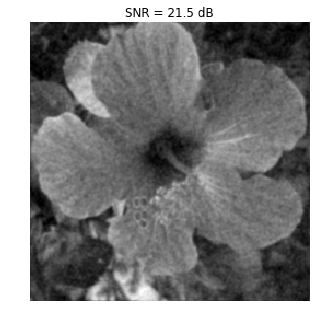In :
## Insert your code here.


## Soft Thresholding SURE¶

In order to enhance the denoising results for piecewise regular signal and image, it is possible to use non-linear thresholding in an orthogonal wavelet basis $\Bb = \{ \psi_m \}_{m}$ where $\psi_m \in \RR^N$ is a wavelet element.

Re-generate a noisy image.

In :
f = f0 + sigma*random.standard_normal((n,n))


The soft-thresholding estimator thus reads $$h_\la(f) = \sum_m s_\la( \dotp{f}{\psi_m} ) \psi_m \qwhereq s_\la(\al) = \max\pa{0, 1-\frac{\la}{\abs{\al}}} \al.$$ It can be conveniently written as $$h_\la = \Ww^* \circ S_\la \circ \Ww$$ where $\Ww$ and $\Ww^*$ are forward and inverse wavelet transform $$\Ww(f) = ( \dotp{f}{\psi_m} )_m \qandq \Ww^*(x) = \sum_m x_m \psi_m,$$ and $S_\la$ is the diagonal soft thresholding operator $$S_\la(x) = ( s_\la(x_m) )_m.$$

Define the wavelet transform and its inverse.

In :
from nt_toolbox.compute_wavelet_filter import *

h_daub = compute_wavelet_filter('Daubechies',4)
W  = lambda f1: perform_wavortho_transf(f1,0,+1,h_daub)
Ws = lambda x: perform_wavortho_transf(x,0,-1,h_daub)


Display the wavelet transform $\Ww(f_0)$ of the original image.

In :
plt.figure(figsize = (10,10))
plot_wavelet(W(f0), 1)
plt.show()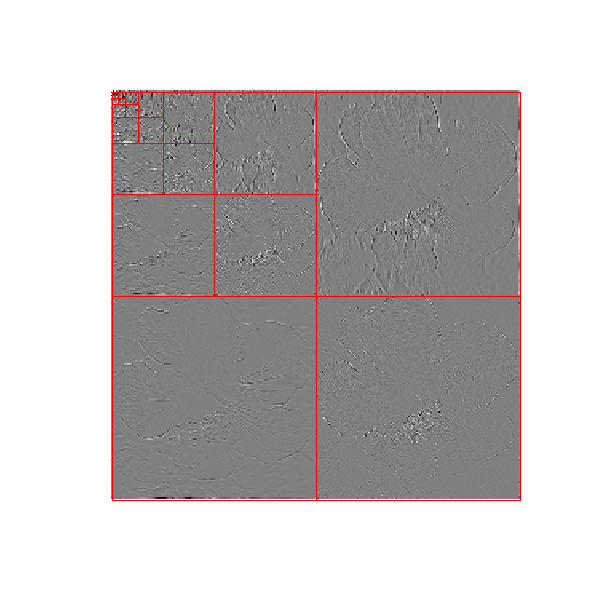Define the soft thresholding operator.

In :
S = lambda x,lambd: np.maximum(np.zeros(list(np.shape(x))), 1-lambd/np.maximum(1e-9*np.ones(list(np.shape(x))),abs(x)))*x


Define the denoising operator.

In :
h = lambda f1,lambd: Ws(S(W(f1), lambd))


Example of denoising result.

In :
lambd = 3*sigma/2

plt.figure(figsize = (5,5))
imageplot(clamp(h(f,lambd)))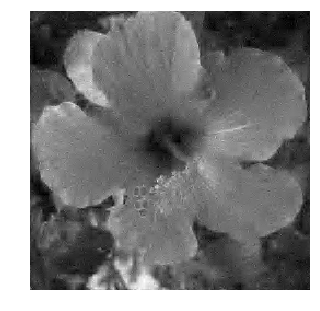Since $Ww$ is an orthogonal transform, one has $$\text{df}(f) = \text{div}( S_\la )( \Ww(f) ) = \sum_m s_\la'( \dotp{f}{\psi_m} ) = \norm{\Ww(h(f))}_0$$ where $s_\la'$ is the derivative of the 1-D function $s_\la$, and $\norm{\cdot}_0$ is the $\ell^0$ pseudo-norm $$\norm{x}_0 = \abs{ \enscond{m}{x_m \neq 0} }.$$

To summarize, the degree of freedom is equal to the number of non-zero coefficients in the wavelet coefficients of $h(f)$.

In :
df = lambda hf,lambd : np.sum(abs(W(hf)) > 1e-8)


We can now define the SURE operator, as a function of $f, h(f), \lambda$.

In :
SURE = lambda f,hf,lambd: -N*sigma**2 + linalg.norm(hf-f, 'fro')**2 + 2*sigma**2*df(hf, lambd)


Exercise 4

For a given $\lambda$, display the histogram of the repartition of the quadratic error $\norm{y-h(y)}^2$ and of $\text{SURE}(y)$. Compute these repartition using Monte-Carlo simulation (you need to generate lots of different realization of the noise $W$). Display in particular the location of the mean of these quantities. Hint: you can do the computation directly over the wavelet domain, i.e. consider that the noise is added to the wavelet transform.

In :
run -i nt_solutions/denoisingadv_9_sure/exo4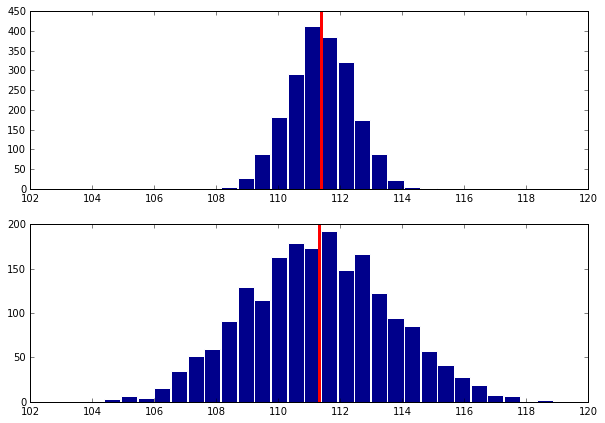In :
## Insert your code here.


Exercise 5

Compute, for a single realization $f=f_0+w$, the evolution of

$$E(\la) = \text{SURE}_\la(f) \qandq E_0(\lambda) = \norm{f-h_\la(f)}^2$$

as a function of $\lambda$.

In :
run -i nt_solutions/denoisingadv_9_sure/exo5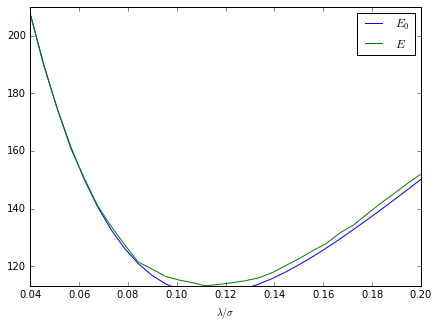In :
## Insert your code here.


Exercise 6

Display the best denoising result $h_{\la^*}(f)$ where $$\la^* = \uargmin{\la} \text{SURE}_\la(f)$$

In :
run -i nt_solutions/denoisingadv_9_sure/exo6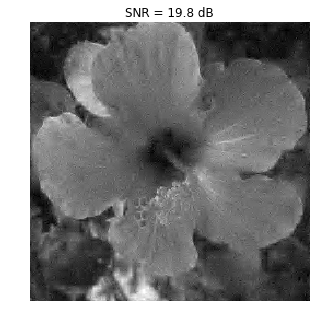In :
## Insert your code here.


## Block-soft Thresholding SURE¶

To improve the result of soft thresholding, it is possible to threshold blocks of coefficients.

We define a partition $\{1,\ldots,N\} = \cup_k b_k$ of the set of wavelet coefficient indexes. The block thresholding is defined as

$$h_\la(f) = \sum_k \sum_{m \in b_k} a_\la( e_k ) \dotp{f}{\psi_m} \psi_m \qwhereq e_k = \sum_{m \in b_k} \abs{\dotp{f}{\psi_m}}^2,$$

where we use the James-Stein attenuation threshold $$a_\la(e) = \max\pa{ 0, 1 - \frac{\la^2}{e^2} }.$$

The block size $q$.

In :
q = 4


A function to extract blocks.

In :
[X,Y,dX,dY] = np.meshgrid(np.arange(1,n-q+2,q),np.arange(1,n-q+2,q),np.arange(0,q),np.arange(0,q))
I = (X + dX - 1) + (Y + dY - 1)*n

for i in range(n//q):
for j in range(n//q):
I[i,j] = np.transpose(I[i,j])

blocks = lambda fw : np.ravel(fw)[I]


A function to reconstruct an image from blocks.

In :
def assign(M,I,H):
M_temp = M
np.ravel(M_temp)[I] = H
return np.reshape(M_temp,(n,n))

unblock = lambda H : assign(np.zeros([n,n]), I, H)


Compute the average energy of each block, and duplicate.

In :
def energy(H):
H_tmp = np.copy(H)
for i in range(n//q):
for j in range(n//q):
H_tmp[i][j] = np.mean(H_tmp[i][j]**2)*np.ones([q,q])
return H_tmp


Threshold the blocks. We use here a Stein block thresholding. All values within a block are atenuated by the same factor.

In :
S = lambda H,lambd : np.maximum(1-lambd**2/energy(H), np.zeros(np.shape(H)))*H


Block thresholding estimator $h_\lambda(f)$.

In :
h = lambda f,lambd: Ws(unblock(S(blocks(W(f)), lambd)))


Example of block denoising.

In :
lambd = 1.1*sigma

plt.figure(figsize = (5,5))
imageplot(clamp(h(f, lambd)))